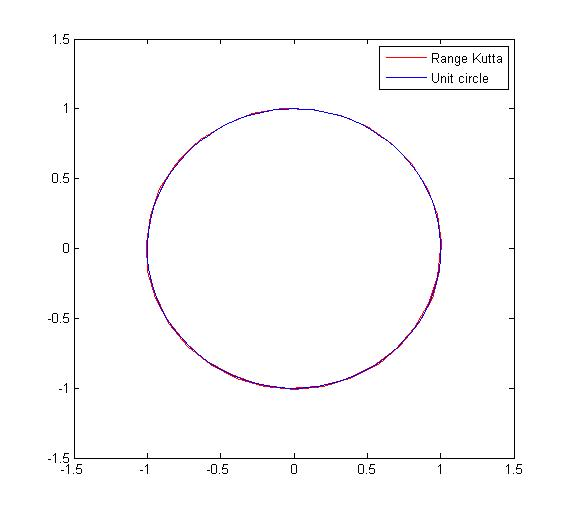Euler VS Runge kutta - Circle - Matlab code | 12CAD.com

# Euler VS Runge kutta – Circle – Matlab code

In the context of this exercise, lets suppose having the two dependent following function: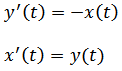With initial conditions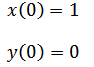The equation of a circle look like the following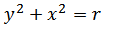where the r is a constant.

If we add our equations at 0, the following will be true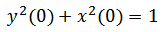We are now going to be using Euler methods and the Runge-Kutta to model a circle of a radius 1 using the above mentioned equations.

## Forward Euler method

Based on the equations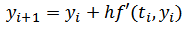We can write the following based on our example.Here is the Matlab code deriving from the above.

```clc;

clear all;

h=0.2; % value of the step, you can vary this to see how it affect the graph

N=51;

x=zeros(1,N);

y=zeros(1,N);

x(1)=1; %initial value x(0)

y(1)=0; %initial value y(0)

%iteration with 50 step

% Forward Euler method

for i=1:N

x(i+1)=x(i)+h*(y(i));

y(i+1)=y(i)-h*(x(i));

end

angle=linspace(0,2*pi); % used to plot the unit circle

plot(x,y,'r',sin(angle),cos(angle),'b')```

And the following plot results from it.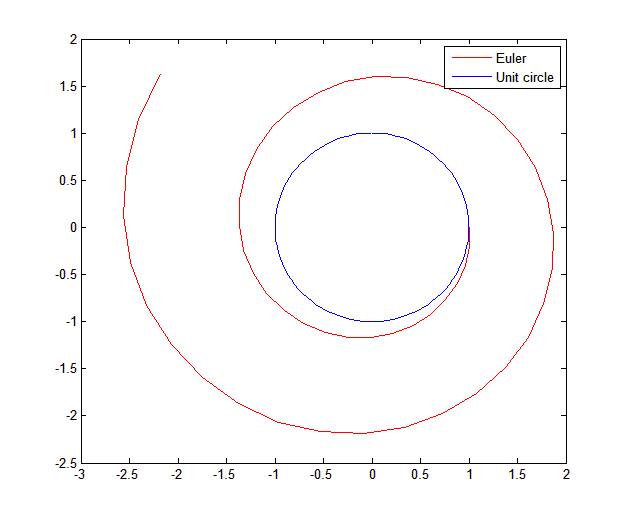## Backward Euler method

Just like the previous method we will start from the formula, then the derivation according to this exercises, the Matlab code and then the graph

Formula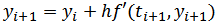Derivation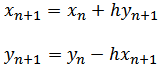we need to arrange the above equation for it to look like the following (substituting and putting n+1 elements on one side and n elements on the other side )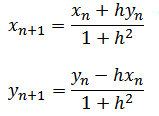Matlab code

```clc;

clear all;

h=0.2;

N=51;

x=zeros(1,N);

y=zeros(1,N);

x(1)=1; %initial value x(0)

y(1)=0; %initial value y(0)

%iteration with 50 step

% Forward Euler method

for i=1:N

x(i+1)=(x(i)+h*(y(i)))/(1+h^2);

y(i+1)=(y(i)-h*(x(i)))/(1+h^2);

end

angle=linspace(0,2*pi); % used to plot the unit circle

plot(x,y,'r',sin(angle),cos(angle),'b')

legend('Euler', 'Unit circle')```

Plot

## Semi-implicit Euler method

Derivation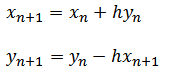Matlab code

```clc;

clear all;

h=0.2;

N=51;

x=zeros(1,N);

y=zeros(1,N);

x(1)=1; %initial value x(0)

y(1)=0; %initial value y(0)

%iteration with 50 step

% Forward Euler method

for i=1:N

x(i+1)=x(i)+h*(y(i));

y(i+1)=y(i)-h*(x(i+1));

end

angle=linspace(0,2*pi); % used to plot the unit circle

plot(x,y,'r',sin(angle),cos(angle),'b')

legend('Euler', 'Unit circle')```

Plot

## Runge Kutta method

Derivation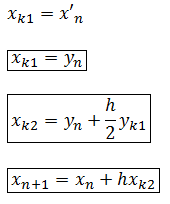Working the same way with y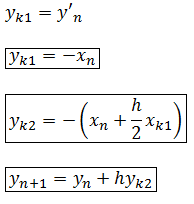Matlab code

``` clc;
clear all;
h=0.2;
N=51;
x=zeros(1,N);
y=zeros(1,N);
x(1)=1; %initial value x(0)
y(1)=0; %initial value y(0)
%iteration with 50 step
% Forward Euler method
for i=1:N
xk1=y(i);
yk1=-x(i);
xk2=y(i)+0.5*h*yk1;
yk2=-(x(i)+0.5*h*xk1);
x(i+1)=x(i)+h*xk2;
y(i+1)=y(i)+h*yk2;
end
angle=linspace(0,2*pi); % used to plot the unit circle
plot(x,y,'r',sin(angle),cos(angle),'b')
legend('Range Kutta', 'Unit circle')```

Plot